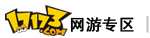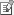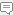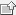| 通行证 注册17173王者世界 > 战术阵型> 正文

2009年03月06日 14:28:32　　　　　　　　　　 【 加入收藏 / 文章投稿 / 截图上传 / 发表评论

混沌之风+大地的鼓动

0转威力:300+(技能等级-1)*2(Lv20=338，Lv40=378，Lv60=418)

1转威力:350+(技能等级-1)*3(Lv20=407，Lv40=467，Lv60=527)

2转威力:400+(技能等级-1)*4(Lv20=476，Lv40=556，Lv60=636)

3转威力:450+(技能等级-1)*5(Lv20=545，Lv40=645，Lv60=745)

4转威力:500+(技能等级-1)*6(Lv20=614，Lv40=734，Lv60=854)

说明

远程系主角技能【混沌之风】与近战系主角【大地的鼓动】的组合技能效果

威力大幅增加，4回合内使敌人全体持续掉血

由于组合技能的等级计算方式暂不清楚，故以上数据仅供参考

混沌之风+大地的鼓动+光之疾走

0转威力:300+(技能等级-1)*10(Lv20=490，Lv40=690，Lv60=890)

1转威力:350+(技能等级-1)*15(Lv20=635，Lv40=935，Lv60=1235)

2转威力:400+(技能等级-1)*20(Lv20=780，Lv40=1180，Lv60=1580)

3转威力:450+(技能等级-1)*30(Lv20=1020，Lv40=1620，Lv60=2220)

4转威力:500+(技能等级-1)*40(Lv20=1260，Lv40=2060，Lv60=2860)

说明

远程系主角技能【混沌之风】、近战系主角【大地的鼓动】和法师系主角【光之疾走】的组合技能效果

攻击敌人全体，威力强大，但效果变为1回合单次攻击技能，不再持续掉血

由于组合技能的等级计算方式暂不清楚，故以上数据仅供参考我要投稿发表/查看评论截图上传
用户： 匿名
史上最强的拼音输入法 下载>>>
评论

## 我要发布Sogou推广服务

404页面_17173.com中国游戏第一门户网站